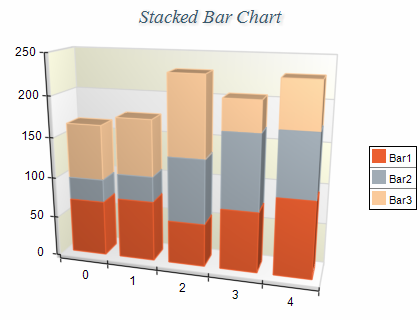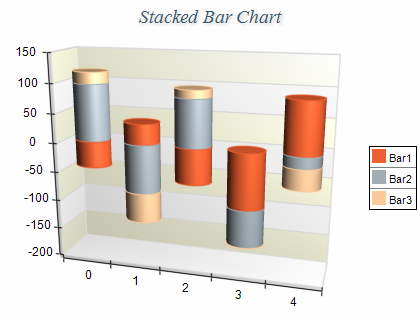﻿ Stacked Bar
Nevron .NET Vision Documentation
Stacked Bar

A Stacked Bar chart displays several series stacked so that there is a single column for each category. The height of each column is determined by the total of all series values for the category. The following figure displays a typical stack bar chart:Figure 1.

Creating the stacked bar chart

Stacked Bar charts are displayed with several NBarSeries objects. The MultiBarMode property of the first bar series must be set to MultiBarMode.Series. The MultiBarMode property of the subsequent bar series must be set to MultiBarMode.Stacked. The following example demonstrates how to create a stack bar chart with two stacks:

C#
Copy Code
```// obtain a reference to the Cartesian chart that is created by default
NCartesianChart chart = (NCartesianChart)chartControl.Charts;

bar1.MultiBarMode = MultiBarMode.Series;
bar2.MultiBarMode = MultiBarMode.Stacked;
```
Visual Basic
Copy Code
```' obtain a reference to the Cartesian chart that is created by default
Dim chart As NCartesianChart = chartControl.Charts(0)

Dim bar1 As NBarSeries = chart.Series.Add(SeriesType.Bar)
Dim bar2 As NBarSeries = chart.Series.Add(SeriesType.Bar)

bar1.MultiBarMode = MultiBarMode.Series
bar2.MultiBarMode = MultiBarMode.Stacked
```

If you want to create a second stacked bar with two stacks behind the stack bar created in the previous sample you have to add the following code:

C#
Copy Code
```NBarSeries bar4 = (NBarSeries)chart.Series.Add(SeriesType.Bar);

bar4.MultiBarMode = MultiBarMode.Series;
bar5.MultiBarMode = MultiBarMode.Stacked;
```
Visual Basic
Copy Code
```Dim bar4 As NBarSeries = chart.Series.Add(SeriesType.Bar)
Dim bar5 As NBarSeries = chart.Series.Add(SeriesType.Bar)

bar4.MultiBarMode = MultiBarMode.Series
bar5.MultiBarMode = MultiBarMode.Stacked
```
Stack Origin
When a bar series is stacked it is always displayed with origin 0, regardless of the values of the UseOrigin and Origin properties.
Stacks with negative values

Stacked bar charts support negative values, which means that if you insert negative values in the Values data series the respective stacks will be piled below the zero. In this case since the values for a certain category can be both positive and negative the chart will display two piles for each category - one for the positive values which grows above the zero and another one for the negative values which grows below the zero.

The following figure demonstrates a typical stack bar chart with negative and positive values:figure 2.

Floating Stacks
One or more Bar series can be stacked over a Float Bar series (NFloatBarSeries) to form floating stack bars. The bars with positive values are stacked on top of the floating bars, while the ones with negative values are stacked under the floating bars.
Formatting Commands

The following formatting commands inherited from the NSeries class have different meaning when a bar series is stacked:

<total> - displays the positive or negative values sum of the values in the current stack. If the current value is negative the negative sum is displayed otherwise the positive sum is displayed.
<cumulative> - displays the sum of the values up to the current stack value.
<percent> - displays the percent contribution of the value to the total pile sum.

Related Examples
Windows forms: Chart Gallery\Bar\Stacked Bar